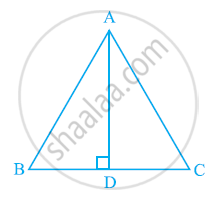Share

# In ΔABC, AD is the perpendicular bisector of BC (see the given figure). Show that ΔABC is an isosceles triangle in which AB = AC. - CBSE Class 9 - Mathematics

ConceptProperties of a Triangle

#### Question

In ΔABC, AD is the perpendicular bisector of BC (see the given figure). Show that ΔABC is an isosceles triangle in which AB = AC.#### Solution

CD = BD (AD is the perpendicular bisector of BC)

∴ AB = AC (By CPCT)

Therefore, ABC is an isosceles triangle in which AB = AC.

Is there an error in this question or solution?

#### APPEARS IN

NCERT Solution for Mathematics Class 9 (2018 to Current)
Chapter 7: Triangles
Ex. 7.20 | Q: 2 | Page no. 123

#### Video TutorialsVIEW ALL 

Solution In ΔABC, AD is the perpendicular bisector of BC (see the given figure). Show that ΔABC is an isosceles triangle in which AB = AC. Concept: Properties of a Triangle.
S